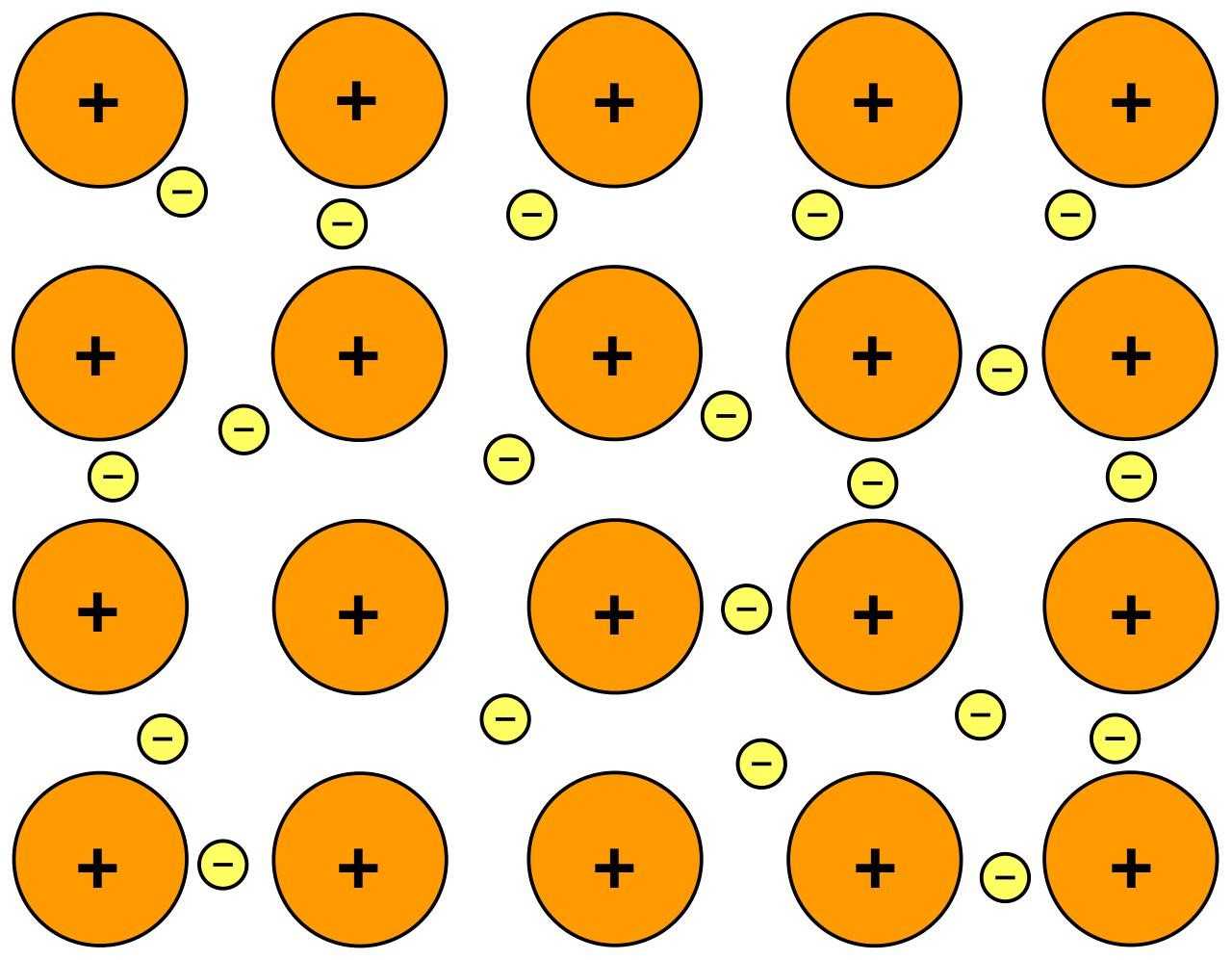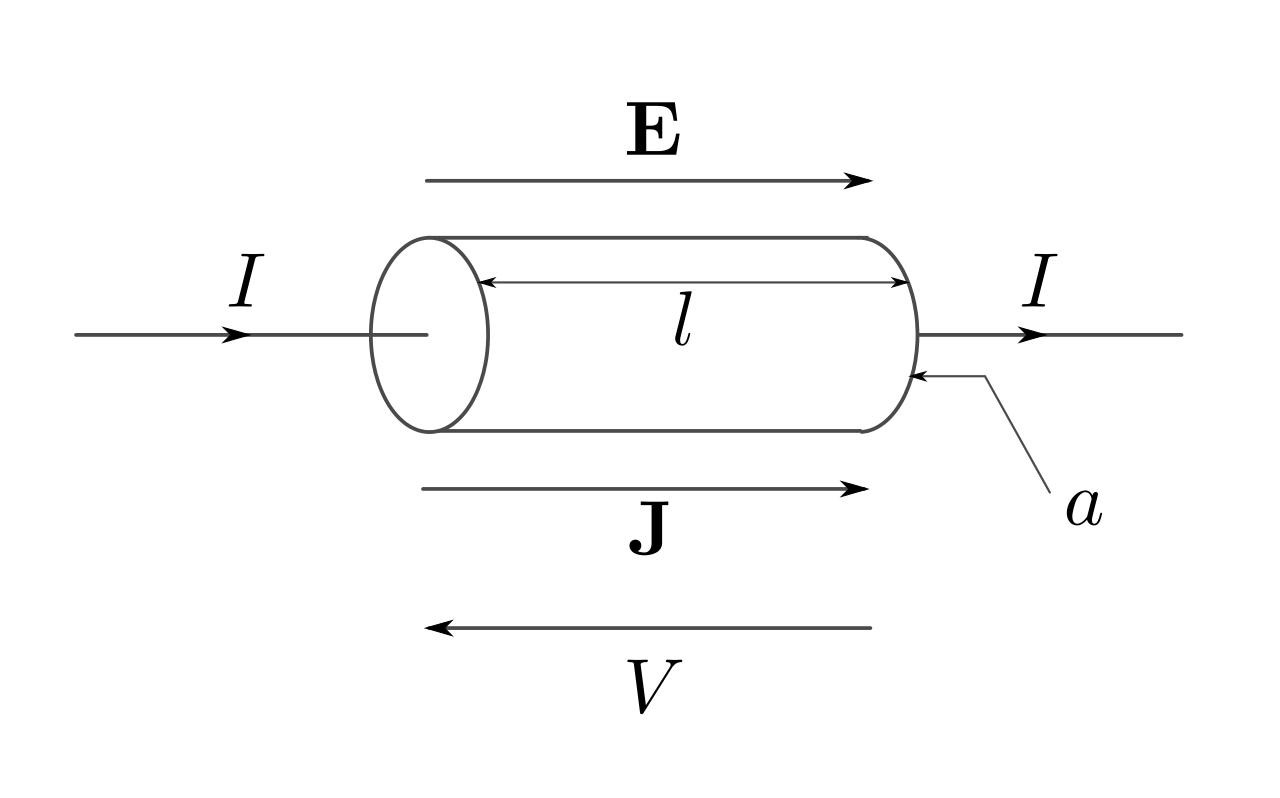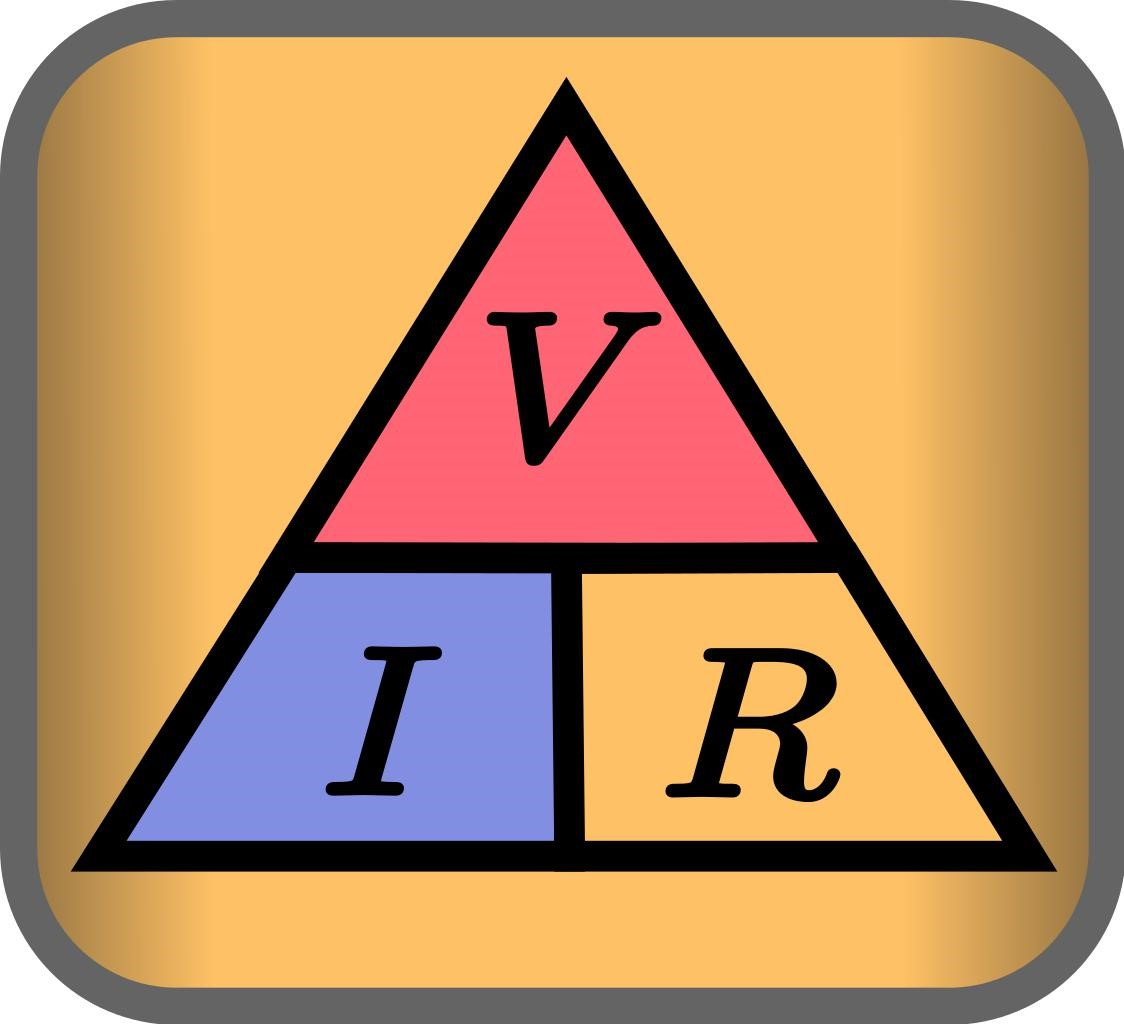Looking for revision notes that are specific to the exam board you are studying? If so, click the links below to view our condensed, easy-to-understand revision notes for each exam board, practice exam question booklets, mindmap visual aids, interactive quizzes, PowerPoint presentations and a library of past papers directly from the exam boards.

# Electrical Resistance

## Key Information & Overview:

• Definitions
• How do Metals conduct Electricity?
• What is Electrical Resistance?
• What are the Factors affecting Electrical Resistance?
• How to measure Electrical Resistance in a Circuit

## Definitions

Metallic Bonding​ is a bonding where positive ions are surrounding by a sea of delocalized electrons.

Current ​is the rate of charge (or charged particles) flowing through. It is measured in amperes, A.

Voltage​ is the electromotive force. In simpler words, it is the force that makes the charged electrons move from one place to another. It is measured in Volts, V.

Electrical Resistance​ is the resistance of the flow of electrons. It is measured in Ohms, Ω.

Resistivity ​is the resisting power of the flow of charged electrons of a metal.

Ohm’s Law ​is a current that states resistance is directly proportional to the voltage and inversely proportional to the current.

## How do metals conduct electricity?

Metals have metallic bonding between its atoms. Metallic bonding occurs when a group of atoms, that form positive charges, come together. The positive ions form a chain and the valence electrons of the atoms become delocalized so that a “sea of electrons” is formed.(This is Metallic Bonding. Positive ions are surrounded by a sea of negative electrons.)

Electrons are negatively charged and they carry the charge with them. As the electrons are delocalized and not fixed in their orbitals, they can carry the charges, move around and pass the charge onto the next electron and thus can conduct electricity.

As metals conduct electricity, the wires in circuits are made of metals, mostly copper. In fact, all the electrical equipment, used in making circuits, is made out of metal.

## What is Electrical Resistance?

Electrical Resistance is what its name suggests. Sometimes, due to some reasons, some charged electrons are stopped and not allowed to go forward. The charged electron flow is resisted and the current decreases.

Even though metal conducts electricity, every metal has some resistive properties that restrict the flow of charged electrons. Some metals are resistive to the current than others.

Electrical Resistance is why the electronics heat up after continuous use. Charged electrons keeping building up at one point and their kinetic energy cause the electronics to heat up.

## What are the Factors Affecting Electrical Resistance?

There are mainly 4 factors that affect the Electrical Resistance:

1. The length of the wire
2. The thickness of the wire
3. The temperature of the wire
4. The material of the wire
• The length of the wire

The shorter the length of the wire, the less the resistance. A long wire has more surface area in contact with the charged particles. Contact causes friction to be produced and friction is a force that opposes the motion. Shorter wires have a shorter surface area thus less resistive force and the charged electrons face less resistance.

• The thickness of the wire

A thicker wire offers less resistance compared to a thinner wire. Think about it in this way: more water will flow out of a pipe with a bigger mouth than a pipe with a smaller mouth. In the same manner, more charged electrons can flow through a thicker wire than a thinner wire which means the electrical resistance of the thicker wire is less.

• The temperature of the wire

The lower the temperature, the less the resistance. We can understand it by thinking about a hyper dog. It will jump and be active and will not listen to you when you tell him to get in the car. Similarly, when the temperature of the wire increases, the kinetic energy of the charged particles increases. Instead of going through the wire, they start colliding with the walls of the wire.

• The material of the wire

Every metal has a different resistance which is called resistivity of the metal. Some metals have a higher resistivity and some metals have lower resistivity. Generally, copper metal has a very low resistivity. This is why wires used in circuits and electrical experiments are made out of copper.(This picture shows different things affecting the electrical resistance.

E = Charged Electrons

I = Rate of flow of Charged Electrons

J = Joules/Energy of the wire (temperature of the wire)

l =lenghth of the wire

A = cross-sectional area (thickness of the wire))

Electrical Resistance of a wire is measured using this formula:

resistance = resistivity × length /area

Question

A copper wire has a length of 0.5 m3and a cross-sectional area of 0.25 m3. The resistivity of copper is 1.7 x10-8Ωm

By using the formula we can calculate the resistance of the copper wire:

resistance = resistivity × length /area

resistance = 1.7 x10-8x 0.5/0.25

resistance = 3.4 x10​-8Ω

## How to measure Electrical Resistance in a Circuit

Ohms law states that the Electrical Resistance is directly proportional to the voltage but inversely proportional to the current.(The formula can be written as V = IR)

It can be reasonably inferred that when:

• When resistance increases, the charged electrons flow decreases and voltage increases.
• When resistance decreases, the charged electron flow increases and thus the voltage decreases.

Question

A circuit a 2 2 volt batteries attached in series. The current flowing through the circuit is 0.2 A. what is the resistance in the circuit?

Solution

First of all, when the batteries are attached in series, their voltages are added. The total voltage of the circuit would be:

2 + 2 = 4 Volts

Secondly, using Ohm’s law we can calculate the resistance.

V = IR

R = V/I

R = 4/0.2

R = 20 Ω

## Summary:

• Resistance is the stopping of the flow of charged electrons.
• Metals can conduct electricity due to their sea of delocalized electrons.
• Smaller wires have less Electrical Resistance than longer wires.
• Thicker wires have less Electrical Resistance than thinner wires.
• Cooler wires have less Electrical Resistance than hotter wires.
• Some metals have lower resistivity than others.
• Resistances of wires is calculated with resistance = resistivity × length /area
• Ohms law states that the Electrical Resistance is directly proportional to the voltage but inversely proportional to the current